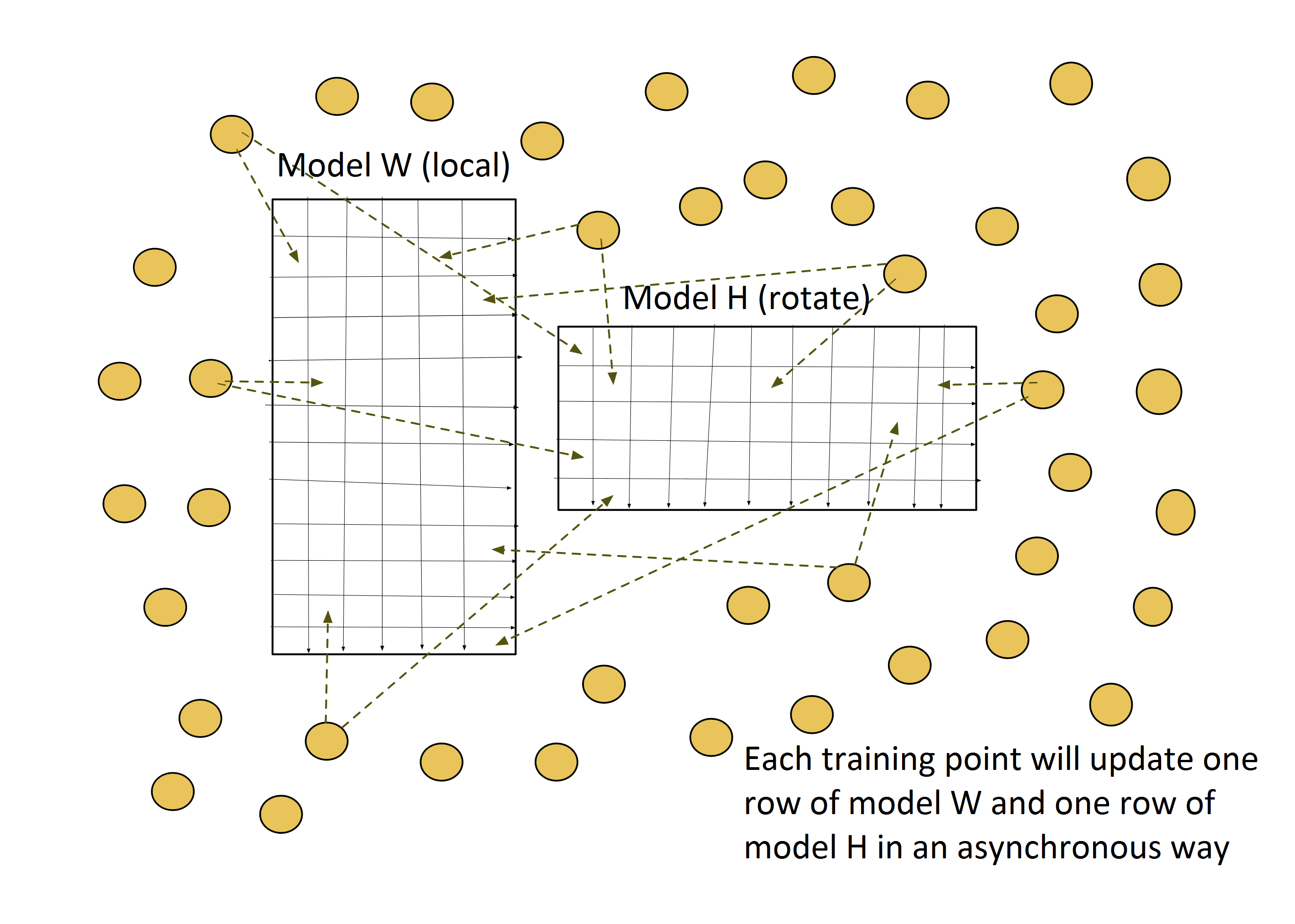## Matrix Factorization based on Stochastic Gradient Descent (MF-SGD)

Matrix Factorization based on Stochastic Gradient Descent (MF-SGD for short) is an algorithm widely used in recommender systems. It aims to factorize a sparse matrix into two low-rank matrices named mode W and model H as follows.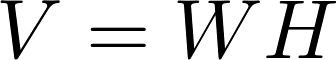The rating Matrix V includes both training data and test data. A learning algorithm uses the training data to update matrices W and H. For instance, a standard SGD procedure will update the model W and H while iterating over each training data point, i.e., an entry in matrix V in the following formula.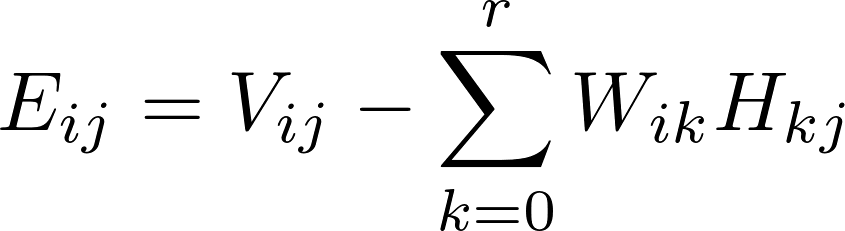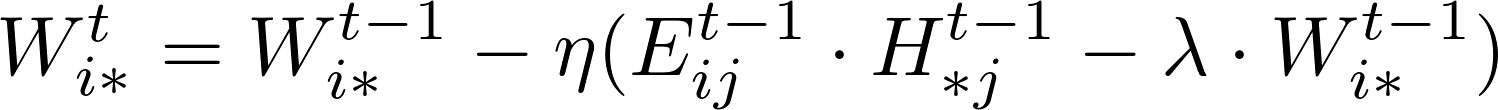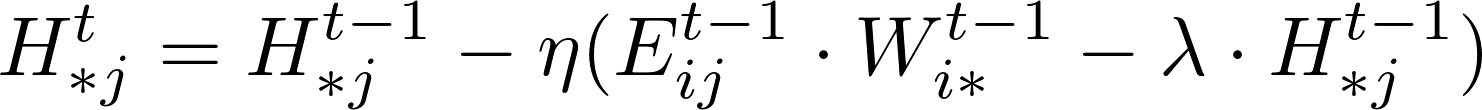After the training process, the test data points in matrix V could be used to verify the effectiveness of the training matrix by computing the RMSE values of the difference## Implementation of SGD within Harp-DAAL Framework

Harp-DAAL-SGD inherits the model-rotation computation model from Harp-SGD. It owns two layers: 1) an inter-mapper layer that decomposes the original MF-SGD problem into different Harp Mappers. 2) an intra-mapper layer that carries out the computation work on local training data in a multi-threading paradigm.

### Inter-Mapper Layout

The training dataset is partitioned by row identities, and each mapper is assigned data points from a group of rows. The model matrix W is also row-partitioned, and each mapper keeps its own local portion of W. The model H is, however, sliced and rotated among all the mappers. Figure 1 shows the inter-mapper layout of Harp-DAAL-SGD.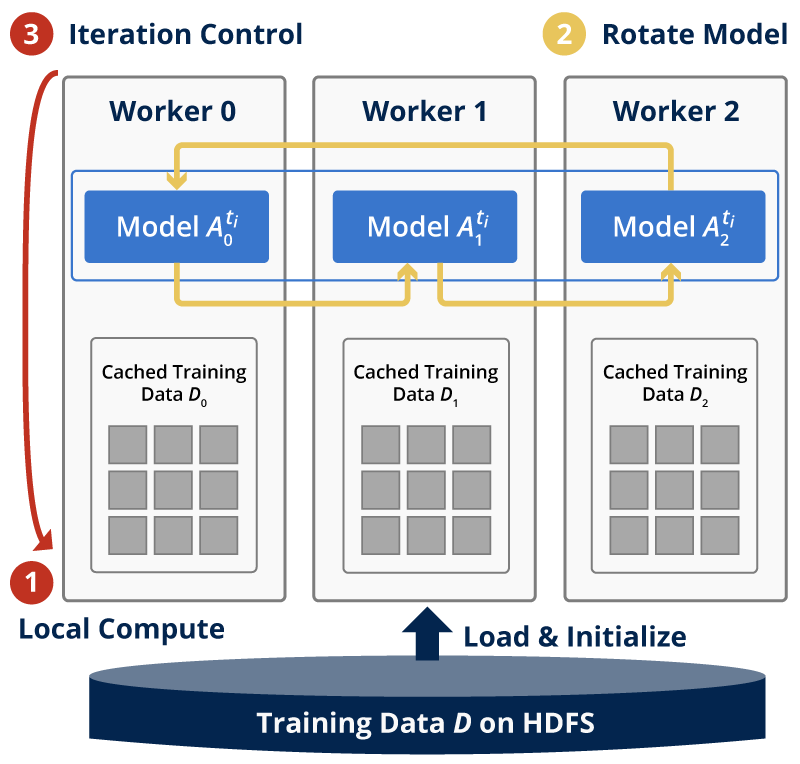### Intra-Mapper Layout

In each iteration, a mapper receives a slice of model H, i.e., a group of columns from matrix H. A procedure will pick out the training data points with column identities from these columns and execute an updating task according to the SGD algorithm. Unlike the model-rotation model, the intra-mapper layer chooses the asynchronous computation model, where each training data point updates its own rows from model matrices W and H without mutual locks.

For the intra-mapper parallel computing, we adopt a hybrid usage of TBB concurrent containers and OpenMP directives.

## A Code Walk Through of Harp-DAAL-SGD

The main body of Harp-DAAL-SGD is the mapCollective function of class SGDDaalCollectiveMapper.

```protected void mapCollective(KeyValReader reader,
Context context) throws IOException, InterruptedException {

try {
runSGD(vFiles, context.getConfiguration(), context);
} catch (Exception e) {
LOG.error("Fail to run SGD.", e);
}
}
```

It first uses the function getVFiles to read in HDFS files. Then, it runs the runSGD to finish the iterative training process. Besides the vFiles, runSGD will also take in the configurations of all the parameters that are required by the training and testing process. The following list includes some of the important parameters.

• r: the feature dimension of model data
• lambda: the lambda parameter in the formula of updating model W and H
• epsilon: the learning rate in the formula of updating model W and H
• numIterations: the number of iterations in the training process
• numModelSlices: the number of pipelines in model rotation

The function runSGD contains several steps as follows:

First it invokes class SGDUtil to load datasets

```//----------------------- load the train dataset-----------------------

```

### Regrouping Training Dataset and Load Data into DAAL

The second step is to re-organize the training dataset among mappers, thus, each mapper will get a portion of data points on a group of rows. Harp provides the following interface for regrouping data:

```regroup("sgd", "regroup-vw", vSetTable, new Partitioner(this.getNumWorkers()));
```

vSetTable is a harp container that consists of different partitions. Here, each partition is an array of training data points with the same row identity. After each mapper gets its proper quote of training data, it starts to load the training data into DAAL’s data container. We use the NumericTable container of DAAL, and its interface receives Java data in a primitive array type. The conversion takes two steps of data copy.

• Copy each partition of vSetTable into a single primitive array of data.
• Copy the primitive array from JVM heap memory into Off-JVM heap memory.

The data copy in the first step is done in parallel by using Java thread package.

``` train_wPos_daal = new HomogenBMNumericTable(daal_Context, Integer.class, 1, workerNumV, NumericTable.AllocationFlag.DoAllocate);
train_hPos_daal = new HomogenBMNumericTable(daal_Context, Integer.class, 1, workerNumV, NumericTable.AllocationFlag.DoAllocate);
train_val_daal = new HomogenBMNumericTable(daal_Context, Double.class, 1, workerNumV, NumericTable.AllocationFlag.DoAllocate);

{
}

for (int q = 0; q<numThreads; q++)
{
train_wPos_daal_sets.get(q),train_hPos_daal_sets.get(q), train_val_daal_sets.get(q)));

}

for (int q=0; q< numThreads; q++) {

try
{
}catch(InterruptedException e)
{
}

}
```

The second step is done inside DAAL codes by using the releaseBlockOfColumnValues function from DAAL’s Java API. This function internally create a direct byte buffer to transfer the data.

```int itr_pos = 0;
{

}
```

### Create Model Matrices and Model Rotator

Two model matrices, W matrix and H matrix, are both the input data and output data. We initialize them with random values, and use them after training to predict new data. Each mapper owns its portion of the whole W matrix, which is local to this mapper. This local W matrix is thus stored at the Off-JVM heap memory space, which is accessible to the DAAL native kernels. We only transfer an array of row identities from Java side into DAAL side, and the initialization is done within DAAL’s kernel before the first iteration.

```//----------------- create the daal table for local row ids -----------------
wMat_size = idArray.size();
wMat_rowid_daal = new HomogenNumericTable(daal_Context, Integer.class, 1, wMat_size, NumericTable.AllocationFlag.DoAllocate);
wMat_rowid_daal.releaseBlockOfColumnValues(0, 0, wMat_size, ids);
```

Unlike the W matrix, the H matrix is rotated among all the mappers multiple times in each iteration. Therefore, we keep one copy at the JVM heap memory and another copy at the native off-JVM heap memory. The conversion of data between the harp table of H model and that of a DAAL container is handled by the rotator class.

```// Create H model
Table<DoubleArray>[] hTableMap = new Table[numModelSlices];
createHModel(hTableMap, numModelSlices, vWHMap, oneOverSqrtR, random);
//create the rotator
RotatorDaal<double[], DoubleArray> rotator = new RotatorDaal<>(hTableMap, r, 20, this, null, "sgd");
rotator.start();
```

As Harp-DAAL-SGD uses two pipelines to overlap the computation and communication work, the data conversion brought by the H model matrix is also likely to be offset by the heavy computation work.

### Local Computation by DAAL Kernels

We implemented the local DAAL codes in the MF-SGD-Distri algorithm of DAAL’s repository. It is highly abstracted as the other DAAL’s algorithms, and the users only need a few lines of codes to invoke it.

```//create DAAL algorithm object, using distributed version of DAAL-MF-SGD
Distri sgdAlgorithm = new Distri(daal_Context, Double.class, Method.defaultSGD);

sgdAlgorithm.input.set(InputId.dataWPos, train_wPos_daal);
sgdAlgorithm.input.set(InputId.dataHPos, train_hPos_daal);
sgdAlgorithm.input.set(InputId.dataVal, train_val_daal);

sgdAlgorithm.input.set(InputId.testWPos, test_wPos_daal);
sgdAlgorithm.input.set(InputId.testHPos, test_hPos_daal);
sgdAlgorithm.input.set(InputId.testVal, test_val_daal);

PartialResult model_data = new PartialResult(daal_Context);
sgdAlgorithm.setPartialResult(model_data);

model_data.set(PartialResultId.presWMat, wMat_rowid_daal);
```

The training and test dataset are imported to sgdAlgorithm as input arguments while the W matrix and H matrix are imported as result arguments. The kernel class is configurable with respect to the precision, the internal algorithm, and so forth. The same sgdAlgorithm could be used in both of the training and test process.

First, we compute the RMSE value before the training process.

```printRMSEbyDAAL(sgdAlgorithm, model_data, rotator, numWorkers, totalNumTestV, wMat_size, 0, configuration);
```

Second, we start the iterative training process loops.

```for (int i = 1; i <= numIterations; i++) {

for (int j = 0; j < numWorkers; j++) {

for (int k = 0; k < numModelSlices; k++) {

//get the h matrix from the rotator
NumericTable hTableMap_daal = rotator.getDaal_Table(k);
model_data.set(PartialResultId.presHMat, hTableMap_daal);

//set up the parameters for MF-DAAL-SGD
sgdAlgorithm.parameter.set(epsilon,lambda, r, wMap_size, hPartitionMapSize, 1, numThreads, 0, 1);

//computation
sgdAlgorithm.compute();

//trigger the rotator after one time of computation
rotator.rotate(k);

}
}
}
```

After each iteration, we can choose to evaluate the training result immediately, or we may evaluate the result after every certain times of training iterations.

## Running the codes

Make sure that the code is placed in the `/harp/experimental` directory. Run the `harp-daal-mfsgd.sh` script here to run the code.

```cd \$HARP_ROOT/experimental
./test_scripts/harp-daal-mfsgd.sh
```

The details of script is here